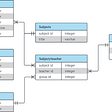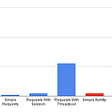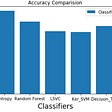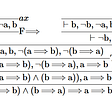# Preconditions

Some dynamic programming problems have a recurrence of this form:

dp(i,j)=min0≤k≤j{dp(i−1,k−1)+C(k,j)}dp(i,j)=min0≤k≤j{dp(i−1,k−1)+C(k,j)}

Where C(k,j)C(k,j) is a cost function and dp(i,j)=0dp(i,j)=0 when j<0j<0.

Say 0≤i<m0≤i<m and 0≤j<n0≤j<n, and evaluating CC takes O(1)O(1) time. Then the straightforward evaluation of the above recurrence is O(mn2)O(mn2). There are m×nm×n states, and nn transitions for each state.

Let opt(i,j)opt(i,j) be the value of kk that minimizes the above expression. If opt(i,j)≤opt(i,j+1)opt(i,j)≤opt(i,j+1) for all i,ji,j, then we can apply divide-and-conquer DP. This is known as the monotonicity condition. The optimal “splitting point” for a fixed ii increases as jj increases.

This lets us solve for all states more efficiently. Say we compute opt(i,j)opt(i,j) for some fixed ii and jj. Then for any j′<jj′<j we know that opt(i,j′)≤opt(i,j)opt(i,j′)≤opt(i,j). This means when computing opt(i,j′)opt(i,j′), we don’t have to consider as many splitting points!

To minimize the runtime, we apply the idea behind divide and conquer. First, compute opt(i,n/2)opt(i,n/2). Then, compute opt(i,n/4)opt(i,n/4), knowing that it is less than or equal to opt(i,n/2)opt(i,n/2) and opt(i,3n/4)opt(i,3n/4) knowing that it is greater than or equal to opt(i,n/2)opt(i,n/2). By recursively keeping track of the lower and upper bounds on optopt, we reach a O(mnlogn)O(mnlog⁡n) runtime. Each possible value of opt(i,j)opt(i,j) only appears in lognlog⁡n different nodes.

Note that it doesn’t matter how “balanced” opt(i,j)opt(i,j) is. Across a fixed level, each value of kk is used at most twice, and there are at most lognlog⁡n levels.

# Generic implementation

Even though implementation varies based on problem, here’s a fairly generic template. The function `compute` computes one row ii of states `dp_cur`, given the previous row i−1i−1 of states `dp_before`. It has to be called with `compute(0, n-1, 0, n-1)`. The function `solve` computes `m` rows and returns the result.

`int m, n;vector<long long> dp_before(n), dp_cur(n);long long C(int i, int j);// compute dp_cur[l], ... dp_cur[r] (inclusive)void compute(int l, int r, int optl, int optr) {    if (l > r)        return;    int mid = (l + r) >> 1;    pair<long long, int> best = {LLONG_MAX, -1};    for (int k = optl; k <= min(mid, optr); k++) {        best = min(best, {(k ? dp_before[k - 1] : 0) + C(k, mid), k});    }    dp_cur[mid] = best.first;    int opt = best.second;    compute(l, mid - 1, optl, opt);    compute(mid + 1, r, opt, optr);}int solve() {    for (int i = 0; i < n; i++)        dp_before[i] = C(0, i);    for (int i = 1; i < m; i++) {        compute(0, n - 1, 0, n - 1);        dp_before = dp_cur;    }    return dp_before[n - 1];}`

# Things to look out for

The greatest difficulty with Divide and Conquer DP problems is proving the monotonicity of optopt. Many Divide and Conquer DP problems can also be solved with the Convex Hull trick or vice-versa. It is useful to know and understand both!

--

--

--

## More from Rajput Ankit

Power corrupts; absolute power corrupts absolutely.

Love podcasts or audiobooks? Learn on the go with our new app.

## Nifty Docker tricks for your CI (vol. 2)## Explore the new SEARCH and CYCLE features in PostgreSQL 14## Structured Query Language (SQL): An Overview## Configure SSH, overclocking, firmware, WiFi, Bluetooth, and VNC for a headless Rasperry Pi 4B with…## Making Concurrent Web API Requests in Python, I Recommend AioHTTP## I was unable to import spacy on Kaggle and here’s how I solved the problem## Flutter plot location on Google Map with Firebase## Rajput Ankit

Power corrupts; absolute power corrupts absolutely.

## Project Garble: Long Audio to Short Text Summarizer## To Reduce Gross NPA and Classify Defaulters Using Shannon Entropy## Biconditional Support for Maslovs Method## How to Record BlueJeans Meeting on Your Desktop — 6 Methods